Note: The other languages of the website are Google-translated. Back to English

## Extract nth word from text string in Excel

If you have a list of text strings or sentences, now, you want to extract the specific nth word from the list as below screenshot shown. This article, I will introduce some methods for solving this job in Excel.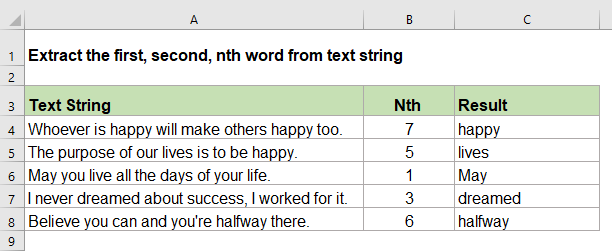#### Extract and get the nth word from text string with formula

To deal with this task, you can use a formula which combines the TRIM, MID, SUBSTITUTE, REPT and LEN functions together. The generic syntax is:

=TRIM(MID(SUBSTITUTE(string," ",REPT(" ",LEN(string))), (N-1)*LEN(string)+1, LEN(string)))
• string: The text string or cell value that you want to extract a word from.
• N: The number of the word you want to extract.

1. Please enter or copy the following formula into a blank cell:

=TRIM(MID(SUBSTITUTE(A2," ",REPT(" ",LEN(A2))), (B2-1)*LEN(A2)+1, LEN(A2)))

Note: In the above formula, A2 is the cell contains the text string that you want to use, B2 is the number of the word that you want to extract, it also can be entered as a number.

2. Then, drag the fill handle down to the cells to apply this formula, and all the specific nth word have been extracted as following screenshot shown: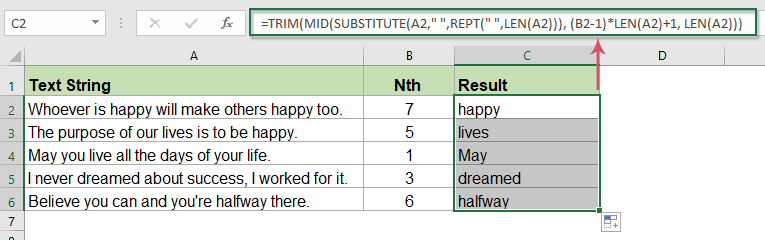##### Explanation of the formula:
• 1. SUBSTITUTE(A2," ",REPT(" ",LEN(A2))):
• REPT(" ",LEN(A2): This REPT function is used to repeat space character by a specific number of times which is the text length of cell A2, this will get a number of space characters;
• SUBSTITUTE(A2," ",REPT(" ",LEN(A2))): The SUBSTITUTE function will replace each space character in cell A2 with a lot of space characters returned by the REPT function, this will get a text string with multiple spaces between each word.
• This part is reorganized as the text argument in the MID function.
• 2. (B2-1)*LEN(A2)+1: This formula will return the starting position of the nth word from the text string in cell A2. It is the start_num argument of the MID function.
• 3. MID(SUBSTITUTE(A2," ",REPT(" ",LEN(A2))), (B2-1)*LEN(A2)+1, LEN(A2)): This MID function extracts the nth word from the text string in cell A2 based on the arguments which are returned by the above formulas.
• 4. TRIM(): This TRIM function removes all extra space characters between the words.

Tips: If there are multiple space characters between words, the above formula will not work correctly, in this case, you should nest another TRIM function inside the SUBSTITUTE function, please apply the below formula:

=TRIM(MID(SUBSTITUTE(TRIM(A2)," ",REPT(" ",LEN(A2))), (B2-1)*LEN(A2)+1, LEN(A2)))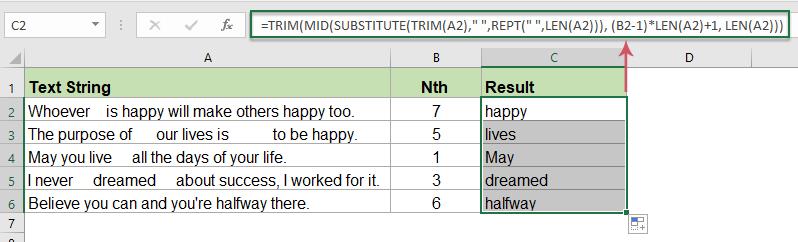#### Extract and get the nth word from text string with User Defined Function

If the above formula is a little difficult for you to understand, here, I will introduce a User Defined Function, with the below code, you can also extract the nth word from a text string. Please do as follows:

1. Press the ALT + F11 keys to open the Microsoft Visual Basic for Applications window.

2. Click Insert > Module, copy and paste the following code in the Module window.

``````Function FindWord(Source As String, Position As Integer)
'Update by Extendoffice
Dim arr() As String
arr = VBA.Split(Source, " ")
xCount = UBound(arr)
If xCount < 1 Or (Position - 1) > xCount Or Position < 0 Then
FindWord = ""
Else
FindWord = arr(Position - 1)
End If
End Function
``````

3. Then, save and close the code module, enter this formula: =findword(A2,B2) into a blank cell where you want to output the result:

Note: In this formula, A2 is the cell contains the text string that you want to use, B2 is the number of the word that you want to extract, it also can be entered as a number.

4. And then, drag the formula into other cells to get the result as you need, see screenshot: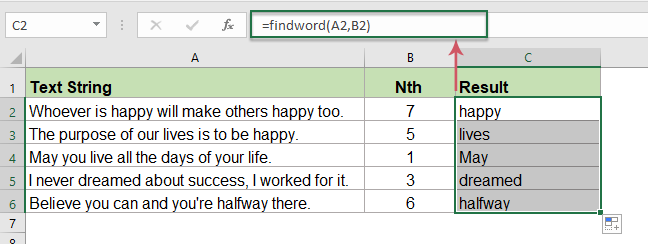#### Extract and get the nth word from text string with a powerful feature

If you have Kutools for Excel, it supports multiple common used formulas for most of the Excel users, with its Extract the nth word in cell utility, you can extract the nth word you specified as quickly as possible. Click to download Kutools for Excel for free trial!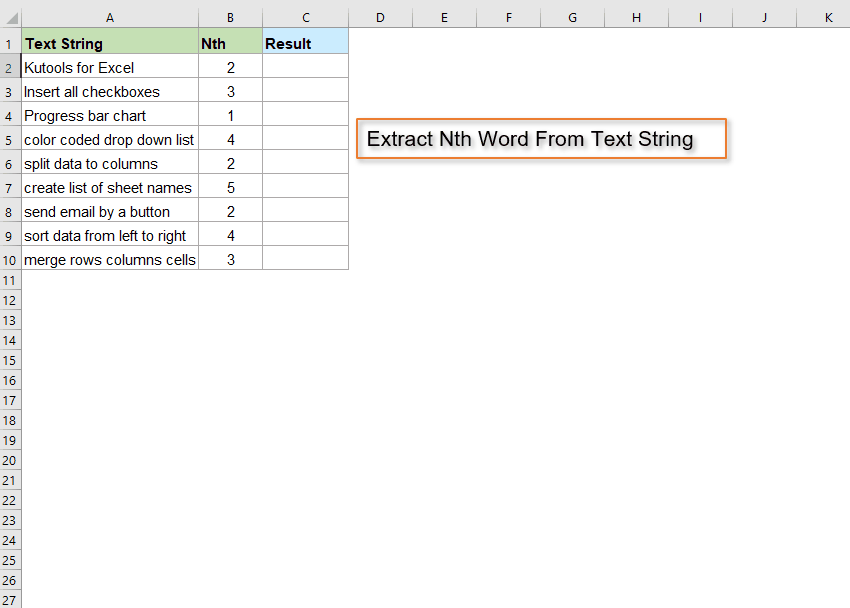#### Relative functions used:

• REPT:
• The REPT function is used to repeat the characters a specified number of times.
• SUBSTITUTE:
• The SUBSTITUTE function replaces text or characters within a text string with another text or characters.
• TRIM:
• The TRIM function removes all extra spaces from text string and only keeps single spaces between words.
• MID:
• The MID function returns the specific characters from the middle of text string.
• LEN:
• The LEN function returns the number of characters in a text string.

#### More articles:

• Extract Last Line Of Text From A Multi-Line Cell
• To extract the last line of text from a text string which is separated by line breaks, normally, there is no direct way for you to solve this. This article, I will introduce a formula to deal with this task in Excel.

### The Best Office Productivity Tools

#### Kutools for Excel - Helps You To Stand Out From Crowd

Would you like to complete your daily work quickly and perfectly? Kutools for Excel brings 300 powerful advanced features (Combine workbooks, sum by color, split cell contents, convert date, and so on...) and save 80% time for you.

• Designed for 1500 work scenarios, helps you solve 80% Excel problems.
• Reduce thousands of keyboard and mouse clicks every day, relieve your tired eyes and hands.
• Become an Excel expert in 3 minutes. No longer need to remember any painful formulas and VBA codes.
• 30-day unlimited free trial. 60-day money back guarantee. Free upgrade and support for 2 years.#### Office Tab - Enable Tabbed Reading and Editing in Microsoft Office (include Excel)

• One second to switch between dozens of open documents!
• Reduce hundreds of mouse clicks for you every day, say goodbye to mouse hand.
• Increases your productivity by 50% when viewing and editing multiple documents.
• Brings Efficient Tabs to Office (include Excel), Just Like Chrome, Firefox, And New Internet Explorer.